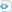One of my favorite .NET control is the ListView. It is very useful for displaying multiple records on a spreadsheet format. Column sorting is one the feature of spreadsheet applications that most of end-users are used to. Unfortunately, this is not readily available as property in VB.NET. The ListView Sorting property only sorts items and not the sub-items so it can’t be use if we want to allow the user to sort your list by any clicked column.

To make your ListView application capable of column sorting, follow these steps:

1. On your existing project, add a new class with following code:

 Source code```Imports System.Collections
Public Class clsListviewSorter
Implements System.Collections.IComparer ' Implements a comparer Implements IComparer
Private m_ColumnNumber As Integer
Private m_SortOrder As SortOrder
Public Sub New(ByVal column_number As Integer, ByVal sort_order As SortOrder)
m_ColumnNumber = column_number
m_SortOrder = sort_order
End Sub
' Compare the items in the appropriate column
Public Function Compare(ByVal x As Object, ByVal y As Object) As Integer Implements IComparer.Compare
Dim item_x As ListViewItem = DirectCast(x, ListViewItem)
Dim item_y As ListViewItem = DirectCast(y, ListViewItem)
' Get the sub-item values.
Dim string_x As String
If item_x.SubItems.Count <= m_ColumnNumber Then
string_x = ""
Else
string_x = item_x.SubItems(m_ColumnNumber).Text
End If
Dim string_y As String
If item_y.SubItems.Count <= m_ColumnNumber Then
string_y = ""
Else
string_y = item_y.SubItems(m_ColumnNumber).Text
End If
' Compare them.
If m_SortOrder = SortOrder.Ascending Then
If IsNumeric(string_x) And IsNumeric(string_y) Then
Return (Val(string_x).CompareTo(Val(string_y)))
ElseIf IsDate(string_x) And IsDate(string_y) Then
Return (DateTime.Parse(string_x).CompareTo(DateTime.Parse(string_y)))
Else
Return (String.Compare(string_x, string_y))
End If
Else
If IsNumeric(string_x) And IsNumeric(string_y) Then
Return (Val(string_y).CompareTo(Val(string_x)))
ElseIf IsDate(string_x) And IsDate(string_y) Then
Return (DateTime.Parse(string_y).CompareTo(DateTime.Parse(string_x)))
Else
Return (String.Compare(string_y, string_x))
End If
End If
End Function
End Class```

2. Declare a private variable on the form where the listview you want to be sorted is located.

3. Then on the listview’s ColumnClick event, add the following code

 Source code```Private Sub ListView1_ColumnClick(ByVal sender As Object, ByVal e As System.Windows.Forms.ColumnClickEventArgs) Handles ListView1.ColumnClick
' Get the new sorting column.
Dim new_sorting_column As ColumnHeader = ListView1.Columns(e.Column)
' Figure out the new sorting order.
Dim sort_order As System.Windows.Forms.SortOrder
If m_SortingColumn Is Nothing Then
' New column. Sort ascending.
sort_order = SortOrder.Ascending
Else ' See if this is the same column.
If new_sorting_column.Equals(m_SortingColumn) Then
' Same column. Switch the sort order.
If m_SortingColumn.Text.StartsWith("> ") Then
sort_order = SortOrder.Descending
Else
sort_order = SortOrder.Ascending
End If
Else
' New column. Sort ascending.
sort_order = SortOrder.Ascending
End If
' Remove the old sort indicator.
m_SortingColumn.Text = m_SortingColumn.Text.Substring(2)
End If
' Display the new sort order.
m_SortingColumn = new_sorting_column
If sort_order = SortOrder.Ascending Then
m_SortingColumn.Text = "> " & m_SortingColumn.Text
Else
m_SortingColumn.Text = "< " & m_SortingColumn.Text
End If
' Create a comparer.
ListView1.ListViewItemSorter = New clsListviewSorter(e.Column, sort_order)
' Sort.
ListView1.Sort()
End Sub```

There you have it, test your listview application and it should be sorting by the column clicked.